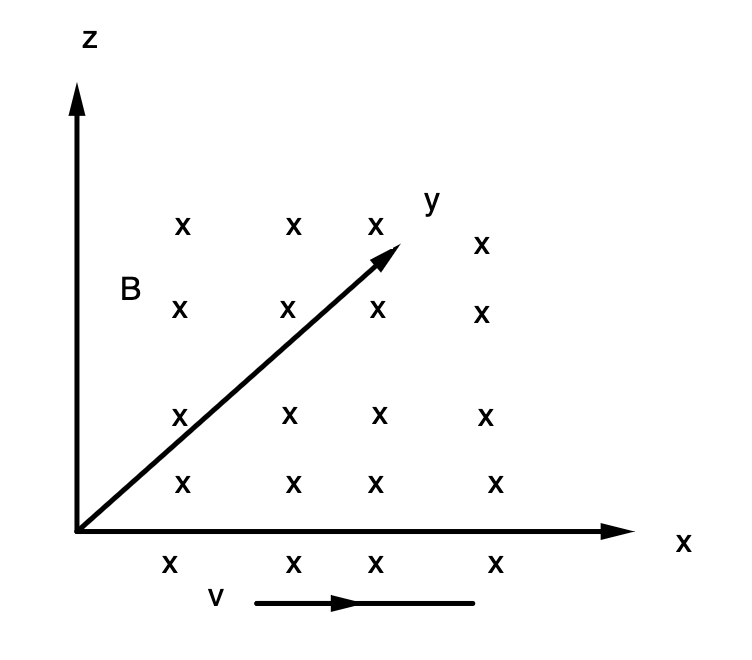Home/Class 12/Physics/

## QuestionPhysicsClass 12

If the magnetic field is parallel to the positive  $$y$$ -axis and the charged particle is moving along the positive  $$x$$ -axis $$($$Fig.$$)$$. Which way would the Lorentz force be for a proton  $$($$ positive charge $$)$$ ?Along $$+z$$-axis.
4.64.6## Solution

Force, $$\vec F=q(\vec v \times \vec B)$$
According to screw rule or right-hand thumb rule we can say that, the velocity  $$\vec v$$  of particle is along the  $$x$$ -axis. While  $$\vec B$$  the magnetic field is along the  $$y$$ -axis. So  $$\vec v \times \vec B$$ is along the $$+z$$ -axis. So, for proton the force is along $$+z$$-axis.